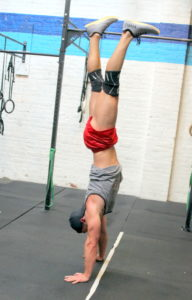## Saturday, September 19, 2020

###Art-Work-Out
as many rounds as possible in 10 minutes:
6x forward burpee over barrier
10x goblet squat @ 45/35-lbs. –or– dumbbell/object squat –or– 20x squat

–rest three minutes–

as many rounds as possible in 10 minutes:
6x plate devil’s press –or– dumbbell/object devil’s press –or– 6x tuck jump
10x pushup

1.—MPH Inside The Box Scores—
Sean F.: 132 + 101 = 233 (45-lbs.)
Andy G.: 132 + 97 = 229 (35-lbs.)
Terry A.: 64 + 34 = 98 (15-lbs.)
Nathan A.: 100 + 97 = 197 (35-lbs.)
Adrian P.: 86 + 65 = 151 (45-lbs.)
Will O.: 160 + 96 = 256 (25-lbs.)
Kate G.: 161 + 115 = 276 (35-lbs.)
Charlie K.: 137 + 84 = 221 (35-lbs.).
Dianna G.: 115 + 98 = 231 (15-lbs.)
Melody F.: 181 + 92 = 273 (35-lbs.)
Chris T.: 142 + 85 = 227 (45-lbs.)
Colin S.: 98 + 70 = 168 (45-lbs.)
Luke B.: 134 + 97 = 231 (25-lbs.)
David C.: 134 + 75 = 209 (25-lbs.)
Travis H.: 114 + 97 = 211 (45-lbs.)
Daniel H.: 120 + 84 = 204 (45-lbs.)
Adrienne V.: 130 + 88 = 218 (25-lbs.)
Andrew B.: 128 + 80 = 208 (25-lbs.)
Amr B.: 80 + 84 = 164 (15-lbs.)
Chris P.: 144 + 98 = 242 (35-lbs.)
Pete T.: 144 + 94 = 238 (35-lbs.)
Yao Z.: 149 + 80 = 229 (35-lbs.)
Tommy H.: 164 + 160 = 324 (45-lbs.)
Sean Q.: 148 + 132 = 280 (45-lbs.)
Josh M.: 134 + 113 = 247 (45-lbs.)
Tarek K.: 133 + 84 = 217 (35-lbs.)
Seth B.: 116 + 84 = 200 (25-lbs.)
Rich H.: 166 + 145 = 311 (45-lbs.)
Bryan M.: 150 + 112 = 262 (45-lbs.)
Sean G.: 178 + 130 = 308 (45-lbs.)

2.